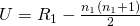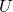# Wilcoxon-Mann-Whitney as an alternative to the t-test

The two sample t-test is one of the most used statistical procedures. Its purpose is to test the hypothesis that the means of two groups are the same. The test assumes that the variable in question is normally distributed in the two groups. When this assumption is in doubt, the non-parametric Wilcoxon-Mann-Whitney (or rank sum ) test is sometimes suggested as an alternative to the t-test (e.g. the Wikipedia page on the t-test), which doesn’t rely on distributional assumptions. But is this necessarily a good ‘replacement’?

The Wilcoxon-Mann-Whitney test
The Wilcoxon-Mann-Whitney (WMW) test consists of taking all the observations from the two groups and ranking them in order of size (ignoring group membership). The ranks of the observations from the first group (it doesn’t matter which group you choose) are then summed, and the test statistic is formed asUnder the null hypothesis that the distribution of the variable in question is identical (in the population) in the two groups, the sampling distribution ofcan be determined (or a normal approximation is invoked) and thus a p-value calculated. The test is available in most (if not all) statistical packages.

What hypothesis is WMW testing?
If WMW is to be used as an alternative to the two sample t-test (for example because the normality assumption made by the latter is in doubt), it would seem a reasonable requirement that it ought to be testing the same ‘thing’. What null and alternative hypotheses is WMW testing? Although papers or books may present a single set of hypotheses, it turns out the WMW test is valid under a range of different sets of possible null and alternative hypotheses (see this paper by Fay and Proschan). But the commonly stated hypotheses are that the distributions in the two groups are the same (null) vs that the probability that a random observation from group 1 exceeds a random observation from group 2 differs from 0.5 (under the null this probability is 0.5).

As a nice article by Fagerland (freely available here) shows, a statistically significant WMW test can result even when the population means of the variable in question are identical in the two groups (i.e. when the t-test null hypothesis is true). Fagerland demonstrates this empirically by simulating data from gamma and log-normal distributions in which the means and medians are identical in the two groups, but the variability (standard deviation) differed in the two groups. The simulations show that the WMW test rejects the null hypothesis more than 5% of the time in these situations (with the rejection rate depending on the particular setup). Of course there is nothing wrong with this result – the distributions in the two groups are not identical, so the null hypothesis of the WMW test is not true, and we would hope that the WMW test would reject the null.

However, if our objective is to test for equality of means, the WMW would mislead us, since the two groups have identical means (and medians) yet the WMW rejects the null hypothesis more than 5% of the time. The difficulty (in my view) with the WMW test is that if we obtain a statistically significant p-value, it is rather unspecific as to what we have found. We have found evidence against the null of identical distributions in the two groups, but we cannot (without doing further analysis) be more specific as to the way in which the two distributions differ.

More specific interpretations of the WMW test can be given, but only if we are willing, in advance of performing the test (and ideally seeing the data), to assume the possibility of a more restrictive alternative hypothesis. One is the location shift, where under the null the two groups have the same distribution, and under the alternative one group’s distribution is shifted in location (see Perspective 6 on page 10 of the paper by Fay and Proschan). However, these sets of nulls and alternatives do not include the one I am assuming in this post is of interest, namely the null that the means of the two groups is the same, versus the alternative that the means differ.

What to do?
As I described in a previous post, provided the sample size is moderately large, the two-sample t-test is robust to non-normality due to the central limit theorem. Fagerland’s simulation results demonstrate this, with the t-test giving a rejection rate of approximately 5% in the simulation study (in contrast to the WMW, which rejects more than 5% of the time). So the usual t-test (possibly allowing for unequal variances) can usually be used, provided the sample sizes are not too small and the distribution is not extremely skewed.

But what if the sample size is small? One thought is to use a permutation test, based on computing the difference in sample means and permuting the group membership. However, this suffers from the same issue as WMW (see here), and so shouldn’t be used if under the null of equal means we believe it is possible for the group’s distributions to differ in other respects.

My personal approach, if I were worried about the normality assumption and the sample size was small, would be to use bootstrapping and the relationship between confidence intervals and hypothesis tests. Specifically, I would find the (superior) bias corrected and accelerated 95% confidence interval for the difference in means between the groups. If this interval excludes the null value of zero, p<0.05, and otherwise p>0.05. If we want to get the actual p-value, we would need to find at what confidence level the confidence interval just includes zero. Although the justification for the bootstrap technique relies on a large sample argument, it has been shown to often work remarkably well with quite small sample sizes.

A different view
A final note in the interest of balance. In reading online when writing this article I came across the following paper by Sawilowsky, who evidently has a quite different view regarding the choice between WMW and the t-test’. That WMW and the t-test are testing different hypotheses is categorised by Sawilowsky as a “True Statement Irrelevant in Choosing Between the t and Wilcoxon” test. Why the fact that the hypotheses being tested differs between the two is irrelevant is not clear to me – if anyone can enlighten me please do so by adding a comment to this page.

For more reading on WMW, the t-test, and its normality assumption, in addition to the previously mentioned papers, I’d also recommend looking at this very readable paper by Lumley and colleagues.

### 7 thoughts on “Wilcoxon-Mann-Whitney as an alternative to the t-test”

1.Assuming independence of observations and non crazy-heavy tails, in the plain vanilla t-test, in large samples, what matters is constant variance of the outcomes across the groups. If that’s violated, the plain vanilla t-test won’t work correctly – but the Welch test (a.k.a. unequal variance t test) will work fine.

As Sawilowsky ignores this important distinction, his critique is rather flawed. I’d ignore it.

What to do? (assuming independence, and assuming you want a test in the first place)

If you have large sample, and want to know about differences in means, use the Welch test – it’s quick, valid, and accurate enough. If you have small samples but want to know about differences in means use a permutation test with a measure of the mean as a test statistic; it’s valid, accurate enough, and not a huge effort. If you don’t want to know about differences in means, say what you do want to know about instead, and go from there.

If you don’t have independence or don’t want a test, start over.

2.Thanks for your comment. I may be wrong, but are you sure that the permutation test (using the difference in means as the test statistic) is valid under the null which only specifies that the means of the two distributions are equal (perspective 15 in the paper by Fay and Proschan)?

The permutation test, as you know, permutes the group labels for observations – this is appropriate under a null which assumes equal distributions in the two groups (i.e. not just equal means). But if the null only specifies equal means, this resampling is not consistent with the null.

3.Thanks for giving a balanced view. The answer is found in Pratt, J. W. (1977). Discussion. The Annals of
Statistics, Vol. 5, page 1092. As for metaman’s comment, (a) the Welch/Welch-Aspin/Satterthwaite does NOT work fine – as noted by a plethora of Monte Carlo evidence, (b) no one in their right mind would ever use a rank-based statistic under those conditions, because as quickly as the t test deteriorates, the Wilcoxon self-destructs even more quickly, and (c) note the first line of the citation you gave: “For treatment effects modeled as a shift in location parameter…”

•Many thanks Shlomo. For others who may be interested, the paper and discussion Shlomo referenced can be accessed here. Just to clarify, do you mean that the fact that the hypotheses being tested by the t-test and Wilcoxon tests are not exactly the same is irrelevant is because in real applications we are not really interested in a specific quantity (like the mean) but are more generally interested in some measure of location (or difference in location between two groups)?

4.What is WMH? You switch to that abbreviation but never define it. Did you mean WMW? Thanks for the informative post.

•Apologies – I did mean WMW! I shall correct it. Thanks.

5.I have enjoyed the conversation and thanks for the paper.

I am considering using the Wilcoxon-Mann-Whitney for my two groups of data. I am looking at the mean-grey-intensity (MGI; 8bit; range:0-255)) in dorsal root ganglion neurons. These neurons have a size distribution of small (0-400um), medium (400-800um), and large (800+um) with a frequency distribution that shows one large peak for small to medium (400um) size and then a much smaller peak in the large population (1200um). The MGI is based on the indirect immunohistochemistry and I am looking at potential protein changes seen as an increase or decrease of pixel intensity. We have consistently observed these changes to be in the small to medium populations. I am comparing small, medium, large, and all sizes. I have tested the normality of my data and many of my comparisons are not in a normal distribution. However, I am not sure if the type of data that I am observing would fit a normal distribution. I have from 150-200 data-points (neurons) per group and the size distribution is robust enough to show the two peaks; one large peak at 400um and a smaller one at 1200um.